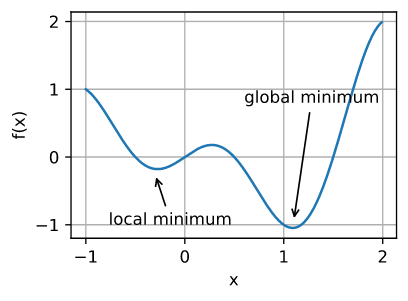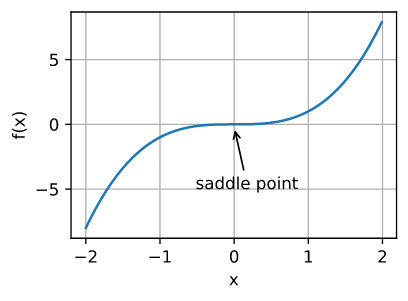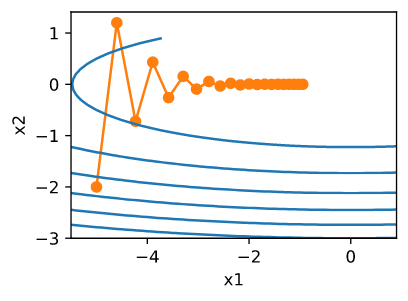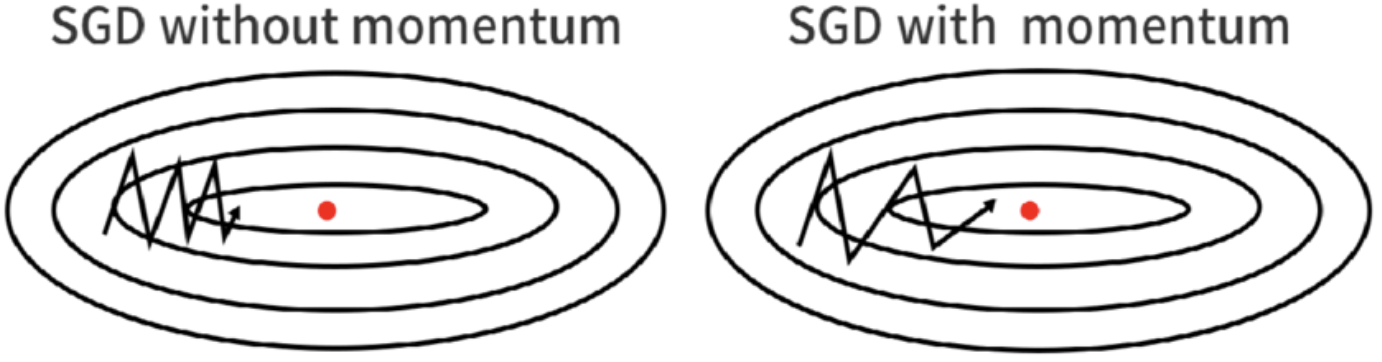# 优化算法

Posted by Masutangu on October 1, 2022

# 优化挑战

## 局部最小值## 鞍点# 优化算法

• 需要全量数据来计算参数更新，当数据集过大时无法放在内存中，影响效率
• 难以支持 online 模式更新，新数据无法很好的融合

• 减少方差，更加稳定
• 能利用矩阵计算提高计算效率

One final but important point regarding SGD is the order in which we present the data to the algorithm. If the data is given in some meaningful order, this can bias the gradient and lead to poor convergence. Generally a good method to avoid this is to randomly shuffle the data prior to each epoch of training.

## 动量法$\mathbf{g_t} = \partial_{\mathbf{w}} \frac{1}{|\mathcal{B_t}|} \sum_{i \in \mathcal{B}\_t} f(\mathbf{x_i}, \mathbf{w_{t-1}})$

$\mathbf{v}_t = \beta \mathbf{v}_{t-1} + \mathbf{g}_{t}$

$\mathbf{w}_t \leftarrow \mathbf{w}_{t-1} - \eta \mathbf{v}_t$AdaGrad 算法通过采用先前观察所得梯度的平方之和作为特征计数器来解决这个问题。定义 $\mathbf{s}_t$ 来累加过去的梯度方差：

\begin{aligned}\mathbf{g}_t & = \partial_{\mathbf{w}} l(y_t, f(\mathbf{x_t},\mathbf{w})), \\ \mathbf{s}_t & = \mathbf{s}_{t-1} + \mathbf{g}_t^2, \\ \mathbf{w}_t & = \mathbf{w}_{t-1} - \frac{\eta}{\sqrt{\mathbf{s}_t + \epsilon}} \cdot \mathbf{g}_t \end{aligned}

# RMSProp

Adagrad 算法将梯度 $\mathbf{g}_t$ 的平方累加成状态矢量 $\mathbf{s}_t = \mathbf{s}_{t-1} + \mathbf{g}_t^2$。 $\mathbf{s}_t$ 会持续增长，几乎上随着算法收敛线性递增。RMSProp 算法采用了类似动量法的做法，即 $\mathbf{s}_t \leftarrow \gamma \mathbf{s}_{t-1} + (1-\gamma) \mathbf{g}_t^2$。

\begin{aligned} \mathbf{v}_t & \leftarrow \beta_1 \mathbf{v}_{t-1} + (1 - \beta_1) \mathbf{g}_t, \\ \mathbf{s}_t & \leftarrow \beta_2 \mathbf{s}_{t-1} + (1 - \beta_2) \mathbf{g}_t^2 \end{aligned}

$\hat{\mathbf{v}}_t = \frac{\mathbf{v}_t}{1 - \beta_1^t}$ $\hat{\mathbf{s}}_t = \frac{\mathbf{s}_t}{1 - \beta_2^t}$

$\mathbf{g}_t' = \frac{\eta \hat{\mathbf{v}}_t}{\sqrt{\hat{\mathbf{s}}_t} + \epsilon}$

$\mathbf{w}_t \leftarrow \mathbf{w}_{t-1} - \mathbf{g}_t'$

# 参考文献